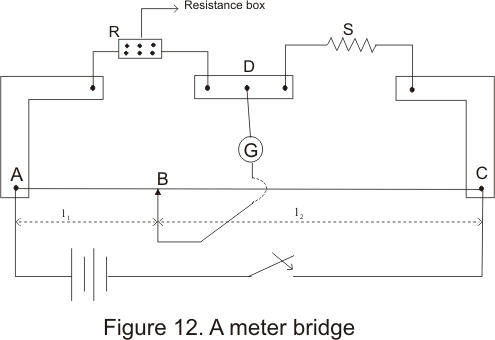# Meterbridge

## Meterbridge (slide wire bridge)

• Meter bridge is based on the principle of wheatstone bridge and it is used to find the resistance of an unknown conductor or to compare two unknown
resistance
• Figure below shows a schematic diagram of a meter bridge• In above figure AC is a 1m long wire made of maganin or constanan having uniform area of cross-section
• This wire is stretched along a scale one a wooden base
• Ends A and C of the wire are screwed to two L shaped copper strips as shown in figure
• A resistance box R and an unknown resistance S are connected as shown in figure
• One terminal of galvanometer is connected to point D and another terminal is joined to a jockey that can be slided on a bridge wire
• when we adjust the suitable resistance of value R in the resistance box and slide this jockey along the wire then a balance point is obtained sat at point B
• Since the circuit now is the same as that of wheatstone bridge ,so from the condition of balanced wheatstone bridge we have
P/Q=R/S
Here resistance P equals
P=ρl1/A
And Q=ρl2/A
where ρ is the resistivity of the material of the wire and A is the area of cross-section of wire
Now P/Q=(ρl1/A)(A/ρl2)=l1/l2